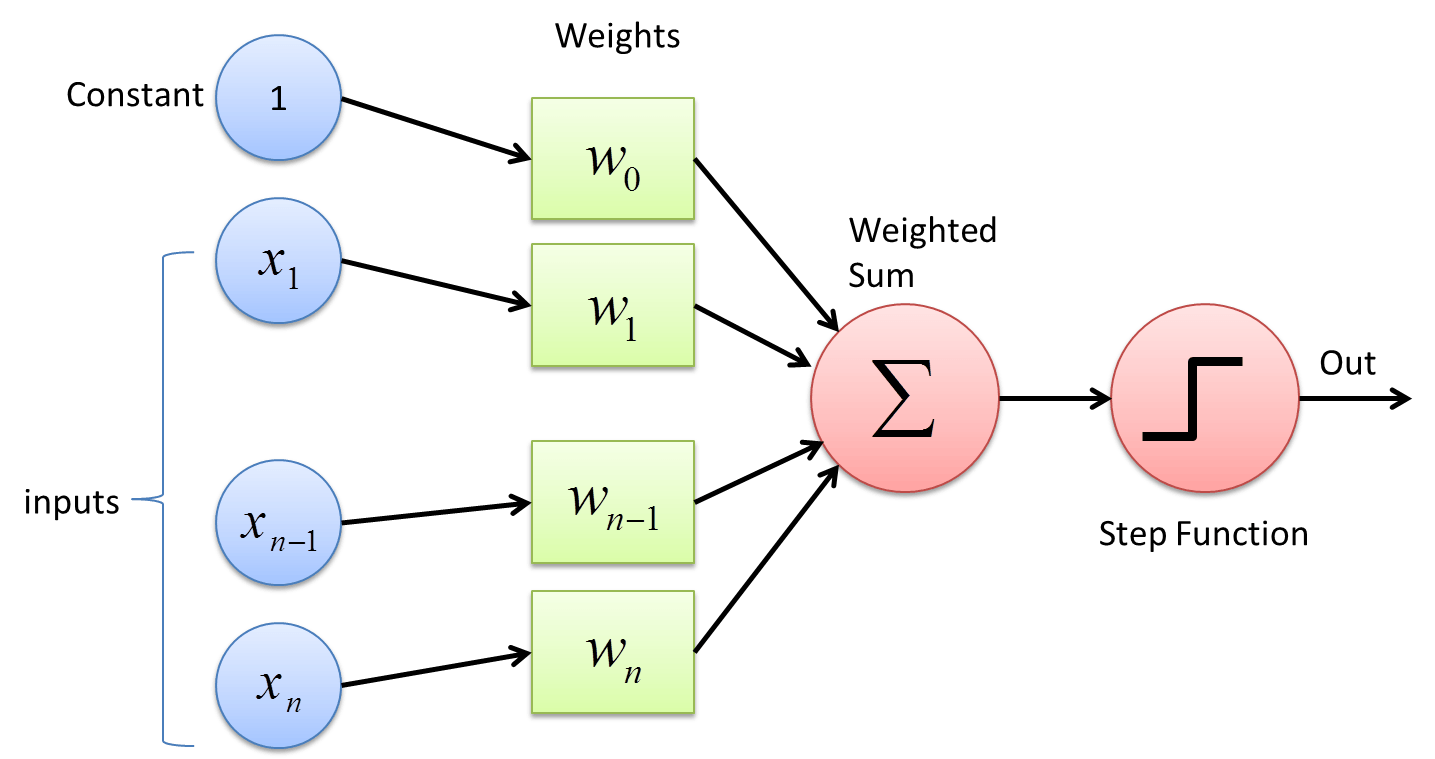# Perceptron

## ﻿What is a Perceptron?

A Perceptron is an algorithm used for supervised learning of binary classifiers. Binary classifiers decide whether an input, usually represented by a series of vectors, belongs to a specific class.

In short, a perceptron is a single-layer neural network. They consist of four main parts including input values, weights and bias, net sum, and an activation function.

## How does a Perceptron work?

The process begins by taking all the input values and multiplying them by their weights. Then, all of these multiplied values are added together to create the weighted sum. The weighted sum is then applied to the activation function, producing the perceptron's output. The activation function plays the integral role of ensuring the output is mapped between required values such as (0,1) or (-1,1). It is important to note that the weight of an input is indicative of the strength of a node. Similarly, an input's bias value gives the ability to shift the activation function curve up or down.Source

### Perceptrons and Machine Learning

As a simplified form of a neural network, specifically a single-layer neural network, perceptrons play an important role in binary classification. This means the perceptron is used to classify data into two parts, hence binary. Sometimes, perceptrons are also referred to as linear binary classifiers for this reason.